Worksheet 2. Ampere force.
Оценка 4.8

# Worksheet 2. Ampere force.

Оценка 4.8
docx
08.05.2020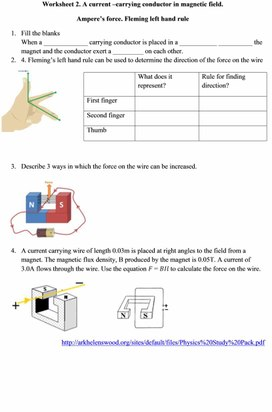Worksheet 2. Ampere force..docx

Worksheet 2. A current –carrying conductor in magnetic field.

Ampere’s force. Fleming left hand rule

1.      Fill the blanks

When a _____________ carrying conductor is placed in a ___________ __________ the magnet and the conductor exert a _________ on each other.

2.      4. Fleming’s left hand rule can be used to determine the direction of the force on the wire

 What does it represent? Rule for finding direction? First finger Second finger Thumb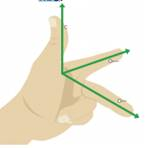3.      Describe 3 ways in which the force on the wire can be increased.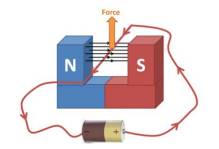4.      A current carrying wire of length 0.03m is placed at right angles to the field from a magnet. The magnetic flux density, B produced by the magnet is 0.05T. A current of 3.0A flows through the wire. Use the equation 𝐹 = 𝐵𝐼𝑙 to calculate the force on the wire.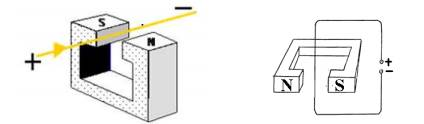http://arkhelenswood.org/sites/default/files/Physics%20Study%20Pack.pdf

5.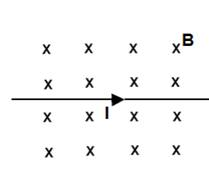A straight long wire carries an electric current to the right. The current is placed in a uniform magnetic field directed into the page. What is the direction of the magnetic force on the current?

A. Left.   B. Right.  C. To the bottom of the page.

D. To the top of the page.  E. Out of the page.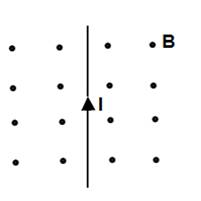6.      A straight long wire carries an electric current to the top of the page. The current is placed in a uniform magnetic field directed out the page. What is the direction of the magnetic force on the current?

A. Left.   B. Right.  C. To the bottom of the page.

D. To the top of the page.  E. Out of the page

7.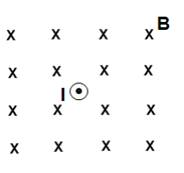A straight long wire carries an electric current out the page. The current is placed in a uniform magnetic field directed into the page. What is the direction of the magnetic force on the current?

A. Left.   B. Right.  C. To the bottom of the page.

D. To the top of the page.  E. There is no magnetic force on the current.

Скачано с www.znanio.ru

## Worksheet 2. A current –carrying conductor in magnetic field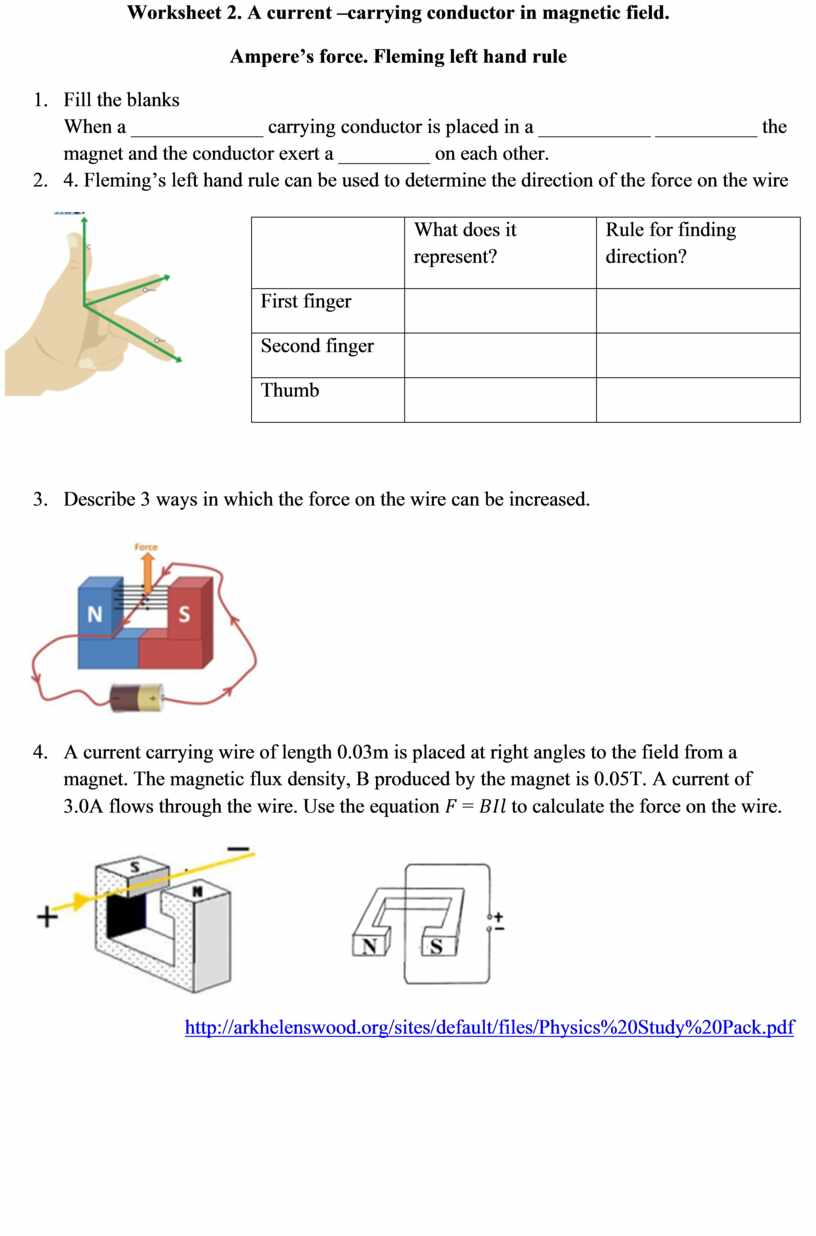## A straight long wire carries an electric current to the rightСкачать файл